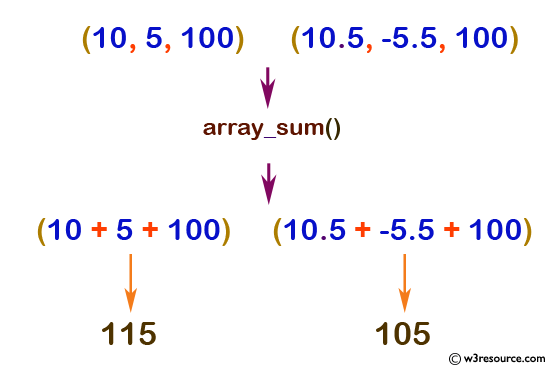# PHP : array_sum() function

## PHP: Calculate the sum of values in an array

The array_sum() function is used to calculate the sum of values in the array.

Version:

(PHP 4 and above)

Syntax:

`array_sum(array_name)`

Parameter:

Name Description Required /
Optional
Type
array_name Specifies the name of the array. Required Array

Return value:

The sum of values.

Value Type: Integer or float.

Example:

``````<?php
\$val1 = array(10, 5, 100);
\$val2 = array(10.5, -5.5, 100);
echo "sum(val1) = ". array_sum(\$val1) . "<br />";
echo "sum(val2) = ". array_sum(\$val2) . "<br />";
?>
``````

Output:

```sum(val1) = 115
sum(val2) = 105```

Pictorial Presentation:View the example in the browser

Practice here online :

PHP Function Reference

Previous: array_slice
Next: array_unique

﻿

## PHP: Tips of the Day

Convert HTML + CSS to PDF

Important: Please note that this answer was written in 2009 and it might not be the most cost-effective solution today in 2019. Online alternatives are better today at this than they were back then.

Here are some online services that you can use:

• PDFShift
• Restpack
• PDF Layer
• DocRaptor
• HTMLPDFAPI
• HTML to PDF Rocket

Have a look at PrinceXML.

It's definitely the best HTML/CSS to PDF converter out there, although it's not free (But hey, your programming might not be free either, so if it saves you 10 hours of work, you're home free (since youalso need to take into account that the alternative solutions will require you to setup a dedicated server with the right software).

Ref : https://bit.ly/384yytr